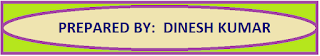### Resource Centre Mathematics

Resource Centre Mathematics Mathematics worksheet, mathematics basic points and formulas, mathematics lesson plan, mathematics multiple choice questions Workplace Dashboard CBSE Syllabus For Session 2023-24 For  :   Classes IX & X    |   Classes XI & XII Watch Videos on Maths Solutions CLASS IX MATHEMATICS FORMULAS &  BASIC CONCEPTS

# Chapter 3 | Matrices

Mathematics assignment for class XII chapter 3, Extra questions with answer key on chapter 3 matrices strictly according to the CBSE syllabus and very useful for examinations.

## Mathematics Assignment On MatricesClass XII Chapter 3

Strictly according to the CBSE Syllabus

Question 1

For the matrix A =  , find   (A - A'), where A' is the transpose of matrix A.

Ans:

Question 2

Construct a 2 x 3 matrix whose elements in the ith row and the jth column are given by   .

Ans:

Question 3

Find a matrix X such that 2A + B + X = 0, where

A =    B =

Ans:

Question 4

If A =  , find k such that   A2 = KA – 2I

Ans:  K = 1

Question 5

Solve for x and y ;    =

Ans: x = 5, y = 3

Question 6

If A =  , find a matrix B such that AB = I

Ans:

Question 7

If B is a skew symmetric matrix, write whether the matrix (ABA') is symmetric or skew symmetric matrix

Ans: Skew symmetric matrix

Question 8

Show that matrix B'AB is symmetric or skew symmetric according as A is symmetric or skew symmetric matrix.

Question 9

Simplify:    +

Ans:

Question 10

If A =   , find x, 0 < x < π/2  , when A + A' = I

Ans:  x = π / 3

Question 11

Let A =  , express A as sum of two matrices such as one is symmetric and other is skew symmetric.

Ansswer

Question 12

If  A =   , find  A2 – 5A +4I and hence find a matrix X such that A2 – 5A + 4I + X = 0

Ans:

Question 13

If A =  , then show that A2 – 4A - 5I = O and hence find A-1

Ans:  A-1 =
Question 14
Find the value of x - y ; if 2  +  =

Question 15:
If A =   and  B =  , then find the value X such that  2A + X = 5B

Question 16

If    =  , Find x

Ans: x = 13

Question 17
If      = 0 , find  x

Answer : x = -2, x = -1
Question 18

If  =  , then find the value of y

Question 19

If  A =  , then Evaluate :  - 4A + 3I

Question 20
If A = [aij] is a square matrix of order 2 such that
then find the value of A2

Question: 21

Question 22

, then  find (AB)T

Question 23
If        then find the value of Z

Question 24
Check whether the following matrix is an orthogonal or not

Answer: Yes it is an orthogonal matrix

Solution Hint

A matrix is said to be orthogonal if   AA’ = I

Question 25
What must be the matrix   X,  if

Question 26
Find x and y , if A =  , B =  and (A + B)2 = A2 + B2
Answer : x = 1, y = 4
Solution Hint
Solve the equation (A +B)2 = A2 + B2 we get
AB + BA = 0
Now in this equation putting the values of matrices A and B and comparing the corresponding terms we get the values of x and y.

Question 27
Verify that the matrix A =   is the inverse of B =
Solution Hint
Find the product AB, If AB = I then A is the inverse of B or B is the inverse of A

Question 28
Three schools X, Y, Z organized a fate (mela) for collecting funds for good victims in which they sold hand-held fans, mats and toys made from recycled material, the sale price of each being Rs 25, Rs 100 and Rs 50 respectively. The following table shows the number of articles of each type sold.

 ⟶   School  Article  ↓ X Y Z Hand-Held Fans Mats Toys 30 12 70 40 15 55 35 20 75

Using matrices, find the funds collected by each school by selling the above articles and the total funds collected.

Ans: Funds collected by school X = ₹ 5450
Funds collected by school Y = ₹ 5250
Funds collected by school Z = ₹ 6625
Total fund collected = ₹ 17325

##1.